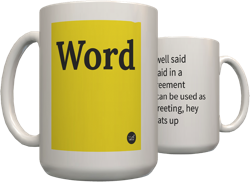2
Hidden scripting language similar to 133t only it attempts to unrelate to words.
a = 2
b = 3
c =
d = q
e = +
f = %
g = p
h = (space)~(space)
i = l
j = )
k = :<
l = I
m = N\
n = /v
o = c
p = 7
q = ?
r = t
s = \$
t = !
u = v
v = ^
w = 1
x = }{
y = -
z = g

? = *
! = #

Whats up?:

13+t = 11~2!5 v7*
by SyINex October 19, 2004Get a 3k mug for your Facebook friend Callisto.
buy the domain for your travel site